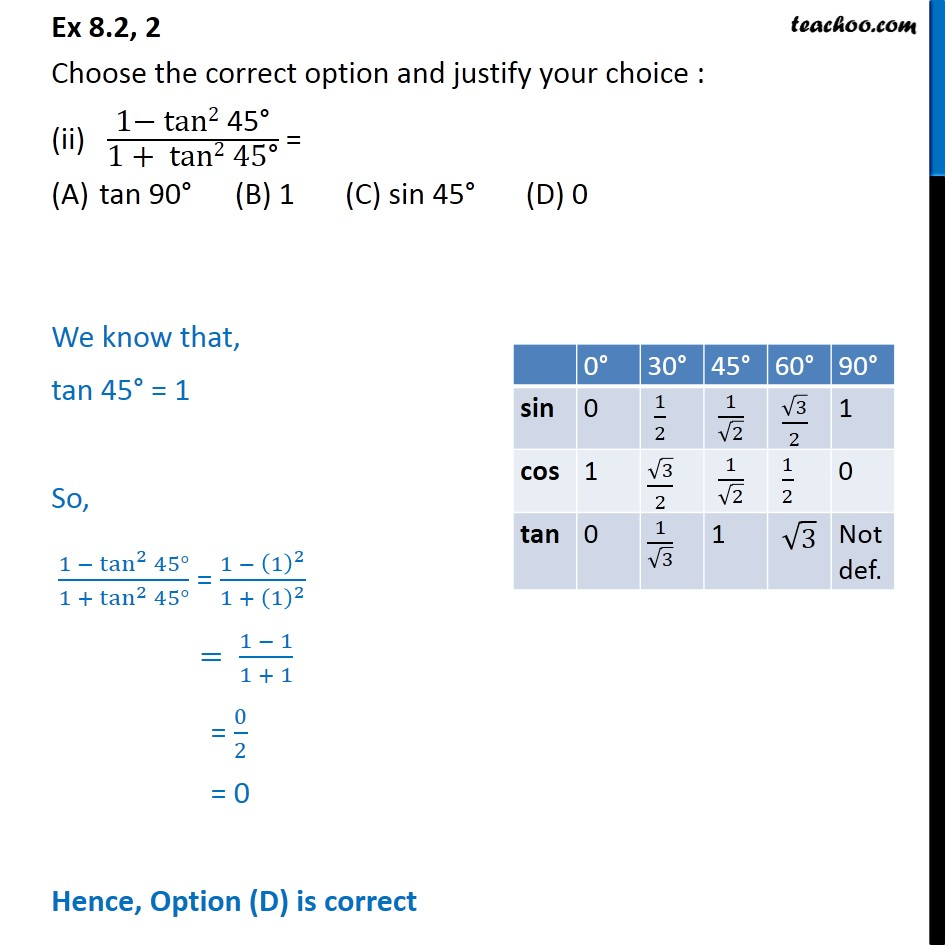1. Chapter 8 Class 10 Introduction to Trignometry (Term 1)
2. Serial order wise
3. Ex 8.2

Transcript

Ex 8.2, 2 Choose the correct option and justify your choice : (ii) "1 tan2 45 " /"1 + tan2 45 " = tan 90 (B) 1 (C) sin 45 (D) 0 We know that, tan 45 = 1 So, (1 tan ^2 45 )/(1 + tan ^2 45 ) = (1 (1)^2)/(1 + (1)^2 ) = (1 1)/(1 + 1) = 0/2 = 0 Hence, Option (D) is correct